# Basic functions - math word problems

#### Number of problems found: 2267

• Lottery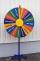The lottery is 60000 elk in which 6200 wins. What is the probability that the purchase of 12 elks won nothing?
• Reciprocal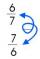Forgiven real numbers, calculate the reciprocal numbers.
• Ratio v2Decrease in the ratio 12:16 number 13.2.
• Six studentsTwo pupils painted the class in four hours. How long will it take for six pupils?
• Selling price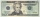Find the selling price. Cost to store: \$50 Markup: 10%
• Playing cards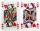How many possible ways are to shuffle 7 playing cards?
• Exponential equationSolve for x: (4^x):0,5=2/64.
• Six on diceWhat is the probability that when throwing two dice will fall at least one six?
• Vans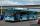In how many ways can 9 shuttle vans line up at the airport?
• Michal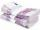Michal has 2.90 per hour. How much does he earn during the week of the summer job?
• Ratio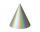The radii of two cones are in the ratio 5.7 Calculate the area ratio if cones have same height.
• Two numbersFind two numbers whose difference and ratio is 2.How many ways can we thread 4 red, 5 blue, and 6 yellow beads onto a thread?
• Word MATEMATIKAHow many words can be created from the phrase MATEMATIKA by changing the letters' order, regardless of whether the words are meaningful?
• PercentCalculate how many % is the number 26.25 less than the number 105.
• Tree trunk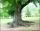Calculate the average tree trunk with a circumference of 149 cm.
• IronIron ore contains 54% iron. How much ore is needed to produce 23 tons of iron?
• ProbabilityHow probable is a randomly selected three-digit number divisible by five or seven?
• Interest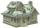What is the annual interest rate on your account if we put 32790 and after 176 days received 33939.2?
• Book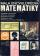To number pages of the thick book was used 4201 digits. How many pages has this book?

Do you have an interesting mathematical word problem that you can't solve it? Submit a math problem, and we can try to solve it.

We will send a solution to your e-mail address. Solved examples are also published here. Please enter the e-mail correctly and check whether you don't have a full mailbox.

Please do not submit problems from current active competitions such as Mathematical Olympiad, correspondence seminars etc...